# GMAT Math : Discrete Probability

## Example Questions

← Previous 1 3 4 5 6 7 8 9

### Example Question #1 : Dsq: Calculating Discrete Probability

Data sufficiency question- do not actually solve the question

A bag of marbles consist of a mixture of black and red marbles. What is the probability of choosing a red marble followed by a black marble?

1. The probability of choosing a black marble first is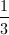.

2. There are 10 black marbles in the bag.

Statement 1 alone is sufficient, but statement 2 alone is not sufficient to answer the question

Statements 1 and 2 together are not sufficient, and additional information is necessary to answer the question

Each statement alone is sufficient to answer the question

Statement 2 alone is sufficient, but statement 1 alone is not sufficient to answer the question

Both statements taken together are sufficient to answer the question, but neither statement alone is sufficient

Both statements taken together are sufficient to answer the question, but neither statement alone is sufficient

Explanation:

From statement 1, we know the probabilty of choosing the first marble. However, since the marble is not replaced, it is impossible to calculate the probability of choosing the second marble. By knowing the information in statement 2 combined with statement 1, we can calculate the total number of marbles initially present.

### Example Question #1 : Discrete Probability

A certain major league baseball player gets on base 25% of the time (once every 4 times at bat).

For any game where he comes to bat 5 times, what is the probability that he will get on base either 3 or 4 times? - Hint – add the probability of 3 to the probability of 4.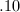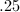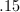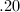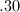Explanation: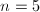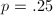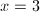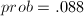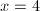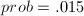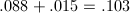Binomial Table

### Example Question #1 : Dsq: Calculating Discrete Probability

A type 1 error (False Alarm or 'Convicting the innocent man') occurs when we incorrectly reject a true null hypothesis.

A type 2 error (failure to detect) occurs when we fail to reject a false null hypothesis.

Which one of the following 5 statements is false?

Note - only 1 of the statements is false.

A) For a given sample size (n=100), decreasing the significane level (from .05 to .01) will decrease the chance of a type 1 error.

B) For a given sample size (n=100), increasing the significane level (from .01 to .05) will decrease the chance of a type 2 error.

C) The ability to correctly detect a false null hypothesis is called the 'Power' of a test.

D) Increasing sample size (from 100 to 120) will always decrease the chance of both a type 1 error and a type 2 error.

E) None of the above statements are true.

A)

B)

C)

E) None of the above statements are true.

D)

E) None of the above statements are true.

Explanation:

Statements A, B, C, and D are all true - so -

The only false statement is E (the statement that declares that A and B and C and D are all false)

### Example Question #1 : Dsq: Calculating Discrete Probability

A marble is selected at random from a box of red, yellow, and blue marbles. What is the probability that the marble is yellow?

1) There are ten blue marbles in the box.

2) There are eight red marbles in the box.

Statement 2 ALONE is sufficient to answer the question, but Statement 1 ALONE is not sufficient.

Statement 1 ALONE is sufficient to answer the question, but Statement 2 ALONE is not sufficient.

BOTH statements TOGETHER are NOT sufficient to answer the question.

BOTH statements TOGETHER are sufficient to answer the question, but NEITHER statement ALONE is sufficient to answer the question.

EITHER Statement 1 or Statement 2 ALONE is sufficient to answer the question.

BOTH statements TOGETHER are NOT sufficient to answer the question.

Explanation:

To determine the probability that the marble is yellow we need to know two things: the number of yellow marbles, and the number of marbles total. The first quantity divided by the last quantity is our probablility.

But the two given statements together only tell us that eighteen marbles are not yellow. This is not enough information. For example, if there are two yellow marbles, the probability of drawing a yellow marble is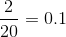. But if there are twenty-two yellow marbles, the probability of drawing a yellow marble is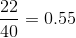Therefore, the answer is that both statements together are insufficient to answer the question.

### Example Question #5 : Discrete Probability

Several decks of playing cards are shuffled together. One card is drawn, shown, and put aside. Another card is dealt. What is the probability that the dealt card is red, assuming the first card is known?

1) The card removed before the deal was red.

2) The cards were shuffled again between the draw and the deal.

Statement 2 ALONE is sufficient to answer the question, but Statement 1 ALONE is not sufficient.

BOTH statements TOGETHER are insufficient to answer the question.

Statement 1 ALONE is sufficient to answer the question, but Statement 2 ALONE is not sufficient.

BOTH statements TOGETHER are insufficient to answer the question.

EITHER statement ALONE is sufficient to answer the question.

BOTH statements TOGETHER are insufficient to answer the question.

Explanation:

To answer this question you need to know two things: the number of red cards left and the number of total cards left. The second statement is irrelevant, as a reshuffle does not change the composition of the deck. The first statement tells you that there is one fewer red card than black cards, but it does not tell you how many of each there are, as you do not know how many decks of cards there were.

And that information, which is not given, affects the answer. For example, if there were four decks, there were 103 red cards out of 207; if there were six decks, there were 155 red cards out of 311. The probabilities would be, respectively,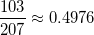and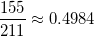a small difference, but nonetheless, a difference.

The correct answer is that both statements together are insufficient to answer the question.

### Example Question #1 : Dsq: Calculating Discrete Probability

Data sufficiency question

What is the probability of choosing a red marble at random from a bag filled with marbles?

1. There are only red and black marbles in the bag

2.of the marbles are black

statements 1 and 2 together are not sufficient, and additional data is needed to answer the question

statement 1 alone is sufficient, but statement 2 alone is not sufficient to answer the question

both statements taken together are sufficient to answer the question, but neither statement alone is sufficient

statement 2 alone is sufficient, but statement 1 alone is not sufficient to answer the question

each statement alone is sufficient

both statements taken together are sufficient to answer the question, but neither statement alone is sufficient

Explanation:

From statement 1, we learn that there are only two different colored marbles in the bag. From statement 2, we learn thatare black which tells us thatare red. Without statement 1, it is impossible to determine if there is another color of marble in the bag.

### Example Question #1 : Dsq: Calculating Discrete Probability

Assume that we are the immortal gods of statistics and we know the following population statistics:

1) average driving speed for women=50 mph with a standard deviation of 12

2) average driving speed for men=45 mph with a standard deviation of 11

We look down from our statistical Mount Olympus and notice that the Earth mortals have randomly sampled 60 women and 65 men in an attempt to detect a significant difference in the average driving speed.

What is the probability that the Earth mortals will properly reject the assumption (i.e. the null hypothesis) that there is no significant difference between the average driving speeds. The Earth mortals have decided to use a 2-tailed 95% confidence test.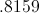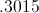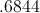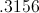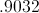Explanation:

standard deviation of the difference between the sample means =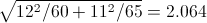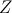at 95% (2-tailed) = 1.96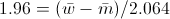the sample difference must be 4 or greater

(note: the probability of the sample difference being -4 or less is so small (4.5 standard deviations) that it will be ignored and we will only consider the probability that the difference is 4 or more.)

the probablity of the sample difference being 4 or greater (knowing that the population difference is 5) =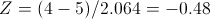the table shows that .3156 lies below -.48, so, .6844 lies above -.48

In English - there is a .6844 probability that the 2 sample means will yield a sample difference that is 1.96 or more standard deviations above 0.

### Example Question #1 : Discrete Probability

In a popular state lottery game, a player selects 5 numbers (on 1 ticket) out of a possible 39 numbers. There are 575,757 possible 5 number combinations.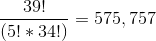So, the odds are 575,757 to 1 against winning.

What are the odds of getting 4 of the 5 numbers correct on 1 ticket?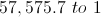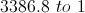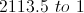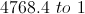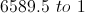Explanation:

575,757 must be divided by -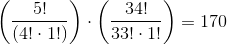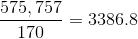### Example Question #1 : Discrete Probability

Your friend at work submits a bold hypothesis. He suggests that the number of sales per day follow the pattern - Monday-10%, Tuesday-10%, Wednesday-10%, Thursday 35% and Friday 35%.

You and he then record the number of sales for the following week: Monday-120, Tuesday-85, Wednesday-105, Thursday-325 and Friday-365.

After viewing the observed data, your friend expresses serious concern regarding his hypothesis.

You can help; you can tell him the probability of the observed data occuring if the hypothesis is true. Hint - Excel ChiTest.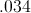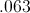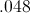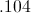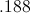Explanation:

Use Excel ChiTest to get the .063 probability. If you are old-fashioned, you can also obtain the Chi-Squared number (8.928) by using ChiInv; but, it is not needed

### Example Question #1 : Discrete Probability

A certain tutor boasts that his 2 week training program will increase a student's score on a 2400 point exam by at least 100 points (4.167%). A 10 student 'before-and-after' study was conducted to validate the claim. The following results were obtained - the 3 columns represent the before, afer and increase numbers for each of the 10 students:

1300 1340 40
1670 1790 120
1500 1710 210
1360 1660 300
1580 1730 150
1160 1320 160
1910 2100 190
1410 1490 80
1710 1880 170
1990 2060 70

Assume the null Hypothesis:

'The average increase is less than 100 points'

What is the highest level of significance (p-value) at which the null hypothesis will be rejected?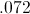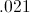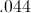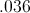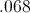Explanation:

Using Excel, the average increase (column 3) is 149 and Standard Deviation of the increases is 76.37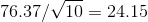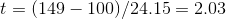Using Excel - a t value of 2.03 for 9 degrees of freedom = .036

← Previous 1 3 4 5 6 7 8 9

### All GMAT Math Resources